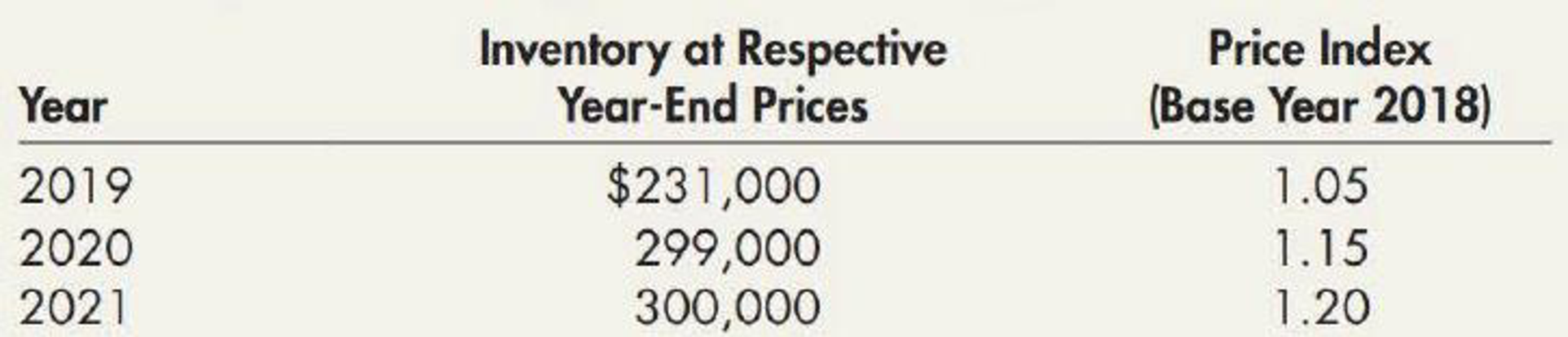Chapter 7, Problem 18E### Intermediate Accounting: Reporting...

3rd Edition
James M. Wahlen + 2 others
ISBN: 9781337788281

#### Solutions

Chapter
Section### Intermediate Accounting: Reporting...

3rd Edition
James M. Wahlen + 2 others
ISBN: 9781337788281
Textbook Problem
208 views

# Dollar-Value LIFO Beistock Company manufactures one product. On December 31, 2018, Belstock adopted the dollar-value LIFO inventory method. The inventory on that date using the dollar-value LIFO inventory method was $200,000. Inventory data for succeeding years are as follows:Required:Compute the ending inventory using the dollar-value LIFO method for 2019, 2020, and 2021. To determine Determine the ending inventory using the dollar value LIFO method for 2019, 2020 and 2021. Explanation Dollar-value LIFO method: In this method, the valuation of inventory is calculated on the monetary value of units instead of quantity of units held. The dollar value LIFO method uses the cost indexes to convert the current cost of inventory to the base year cost. Determine the ending inventory using the dollar value LIFO method for 2019: Ending inventory for the year 2019}=(Inventory at base year(2018) cost )+(Inventory at current year cost )=$200,000+[($220,000$200,000)×1.05]=$200,000+$21,000=$221,000 Determine the ending inventory using the dollar value LIFO method for 2020: Ending inventory for the year 2020}=(Inventory at base year cost for 2019)+(Inventory at current year cost for 2020 )=$221,000+[($260,000$220,000)×1

### Still sussing out bartleby?

Check out a sample textbook solution.

See a sample solution

#### The Solution to Your Study Problems

Bartleby provides explanations to thousands of textbook problems written by our experts, many with advanced degrees!

Get Started

#### Find more solutions based on key concepts